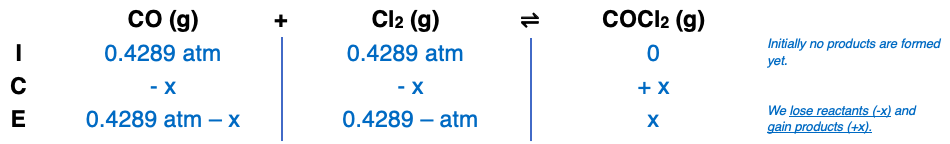# Problem: Carbon monoxide and chlorine gas react to form phosgene: CO(g) + Cl2(g) ⇌ COCl2(g); Kp = 3.10 at 700 KIf a reaction mixture initially contains 326 torr of CO and 326 torr of Cl2, what is the mole fraction of COCl2 when equilibrium is reached?

###### FREE Expert Solution

Step 1

= 0.4289 atm

Step 2Step 3

concentration cannot be negative so we will use

x = 0.1848 atm

88% (120 ratings)###### Problem Details

Carbon monoxide and chlorine gas react to form phosgene: CO(g) + Cl2(g) ⇌ COCl2(g); Kp = 3.10 at 700 K

If a reaction mixture initially contains 326 torr of CO and 326 torr of Cl2, what is the mole fraction of COCl2 when equilibrium is reached?

Frequently Asked Questions

What scientific concept do you need to know in order to solve this problem?

Our tutors have indicated that to solve this problem you will need to apply the ICE Chart concept. You can view video lessons to learn ICE Chart. Or if you need more ICE Chart practice, you can also practice ICE Chart practice problems.

What professor is this problem relevant for?

Based on our data, we think this problem is relevant for Professor Billman's class at Abilene Christian University.